# Lecture 2 | Red Black Tree & B+ Tree¶

## 红黑树¶

### 概念¶

Red Black Tree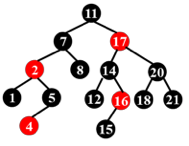Properties of RBTree

@cy's PPT

1. Every node is either red or black.
2. The root is black.
3. Every leaf (NIL) is black.
4. if a node is red, then both its children are black.
5. For each node, all simple paths from the node to descendant leaves contain the same number of black nodes.

ch 老师说，希望我们能把这五条性质熟练记住，怎么可能（逃）

@Wiki
1. Every node is either red or black.
2. All NIL nodes (figure above) are considered black.
3. A red node does not have a red child.
4. Every path from a given node to any of its descendant NIL nodes goes through the same number of black nodes.
@OI Wiki
1. 每一个节点要么是色，要么是色；
2. NIL 节点（空叶子节点）为色；
3. 色节点的子节点必须为色；
4. 从根节点到 NIL 节点的每条路径上的色节点数量相同；

black height, bh

16 号节点的右儿子是一个黑叶子，而这个叶子到根的路径上只有 3 个黑节点，而其他叶子到根都有 4 个黑节点。

or

the proof of the property
1. 首先我们有 $$N \geq 2^{bh}-1$$，也就是 $$bh \leq \log_2 (N+1)$$
2. 然后显然有 $$2 bh(Tree) >= h(Tree)$$

### 操作¶

#### 插入¶

1. 如果 x.p 是黑色的，那么我们不需要做任何调整；
2. 如果 x.p 是红色的，那么我们需要进行调整；
• 此时因为原来的树符合红黑性质，x.p.p 必定存在（红 x.p 不能是根）且必定是黑色的；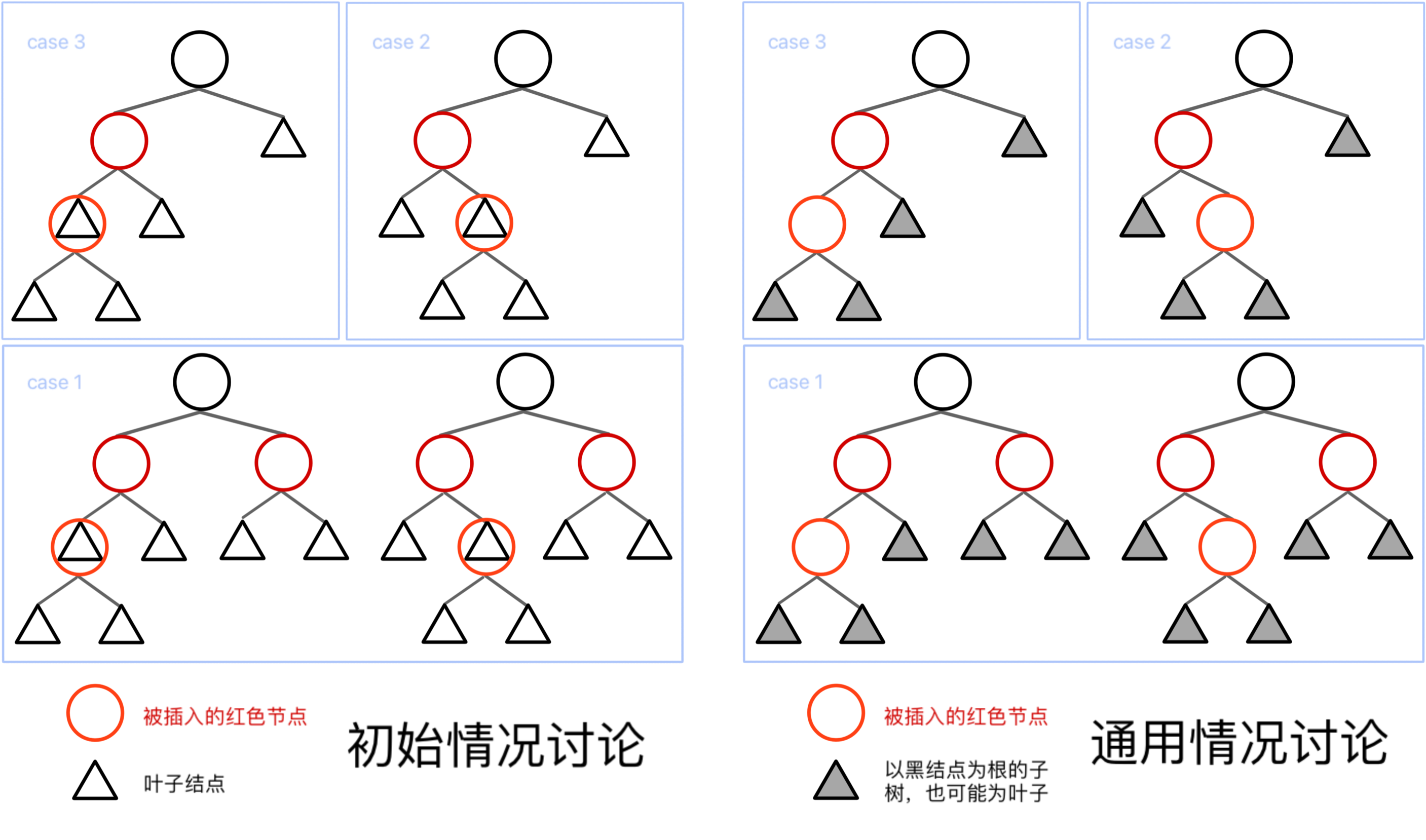Insertion / case 3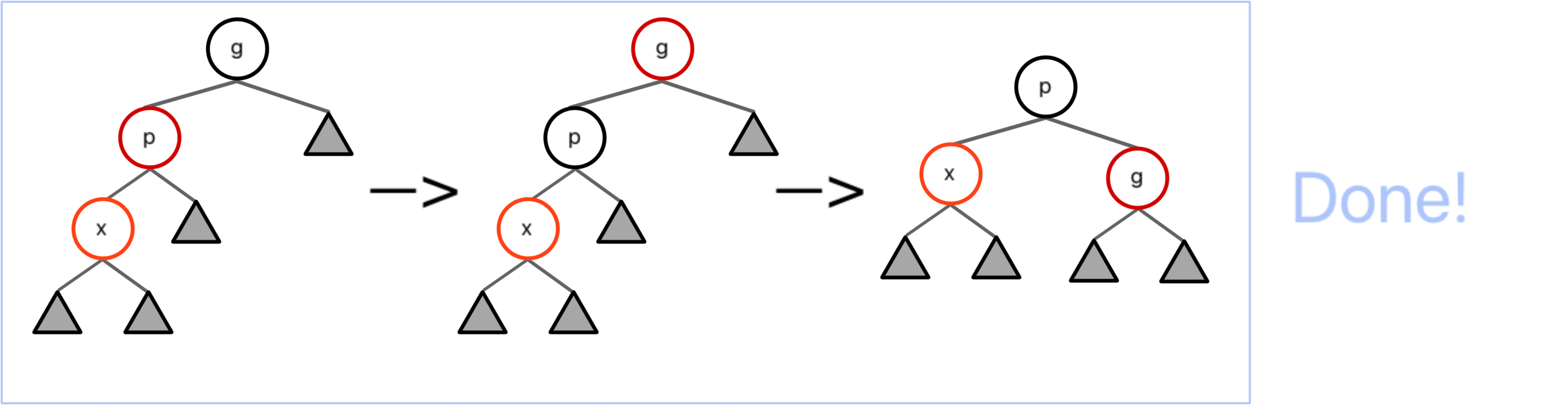Insertion / case 2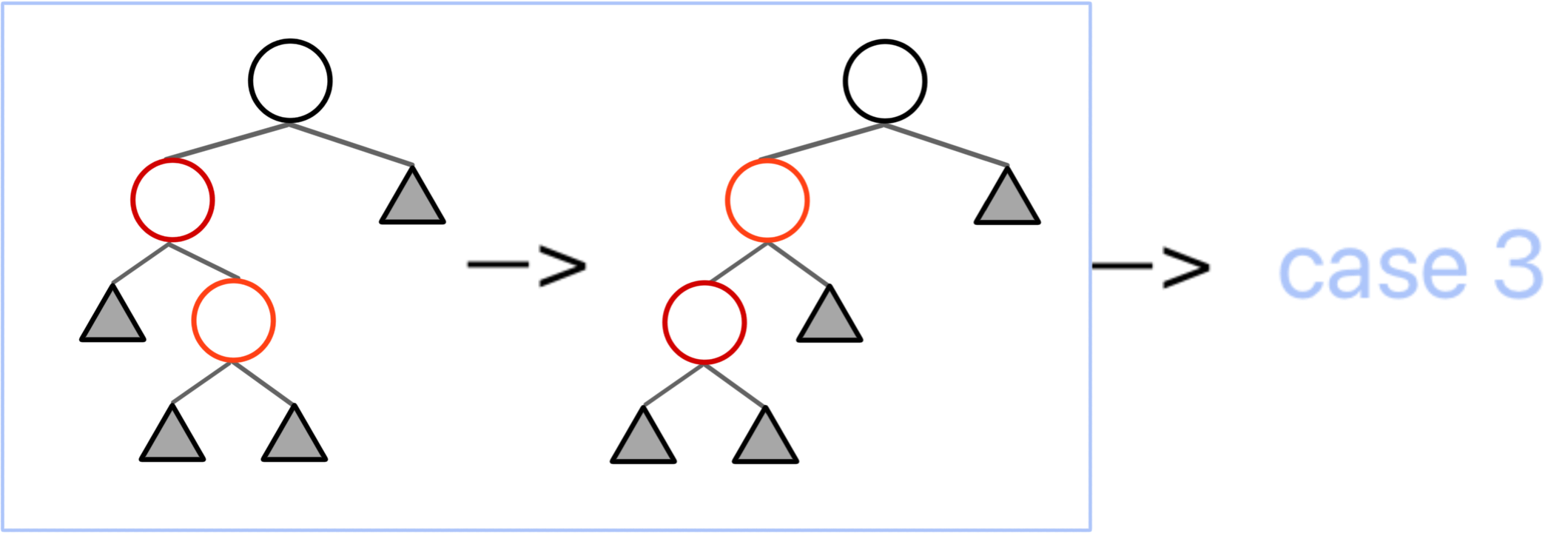Insertion / case 1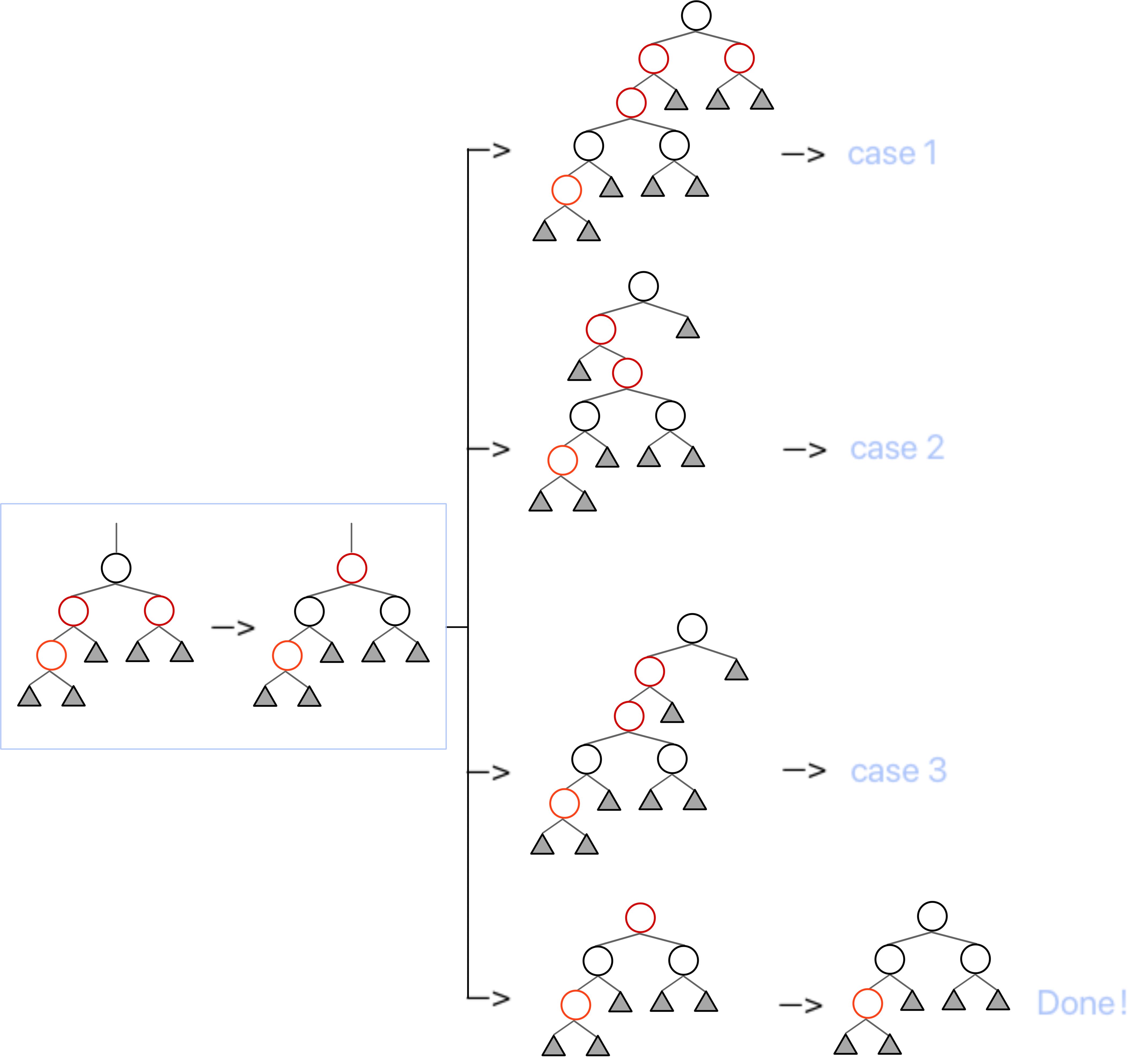graph LR;
A["case 1"]
B["case 2"]
C["case 3"]
D(["finish"])

A ===>|"C"| B --->|"R"| C
A ===>|"C"| A --->|"C"| D
A ===>|"C"| C --->|"C&R"| D

#### 删除¶

• TODO: 这里需要配图讲清楚节点的具体变换到底是怎么样的，尤其是节点颜色变化到底是怎么一回事，之后大概需要重写

my cy's my cy's
case 1 case 2 case 2 case 4
case 3 case 3 case 4 case 1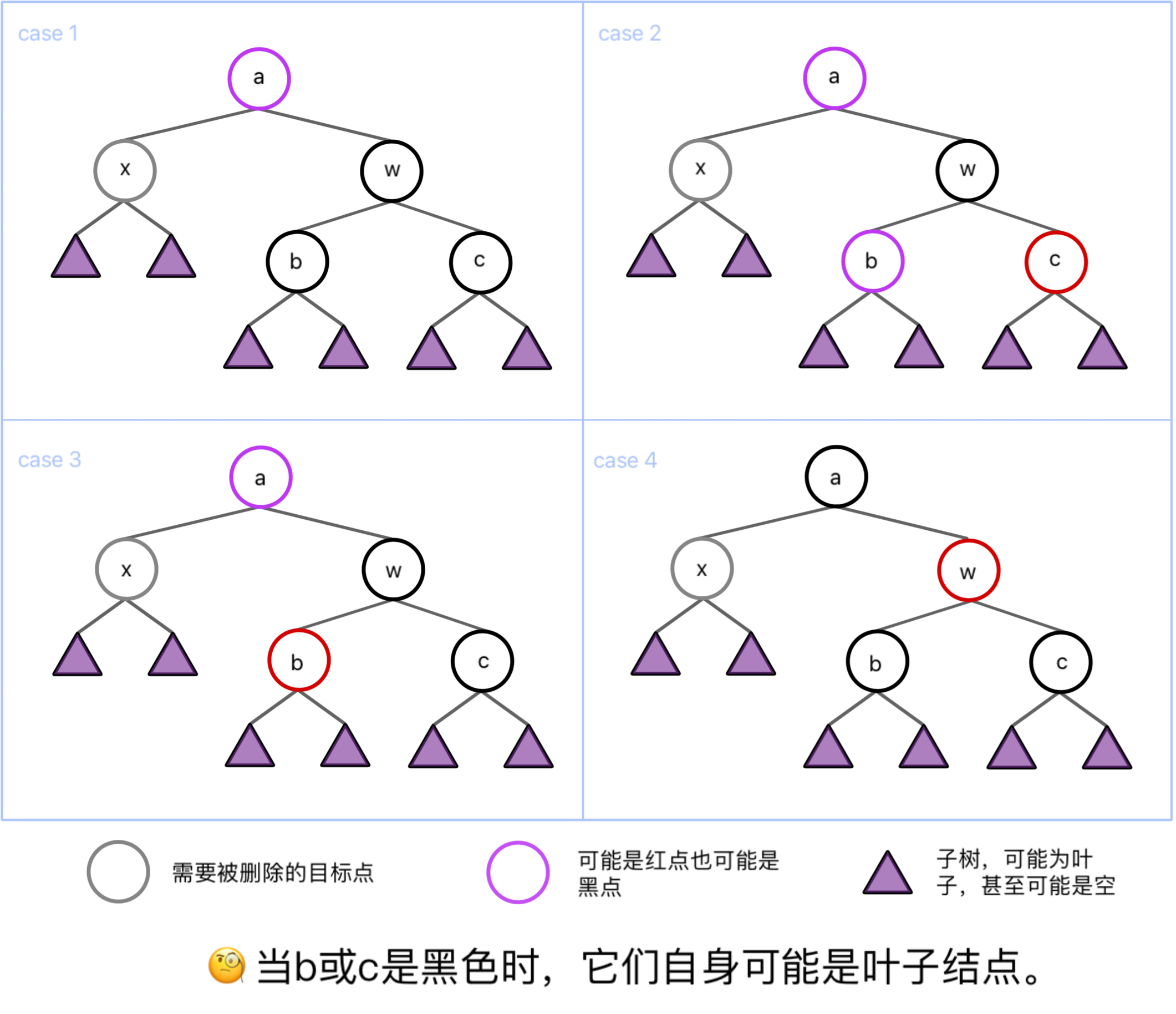Deletion / case 1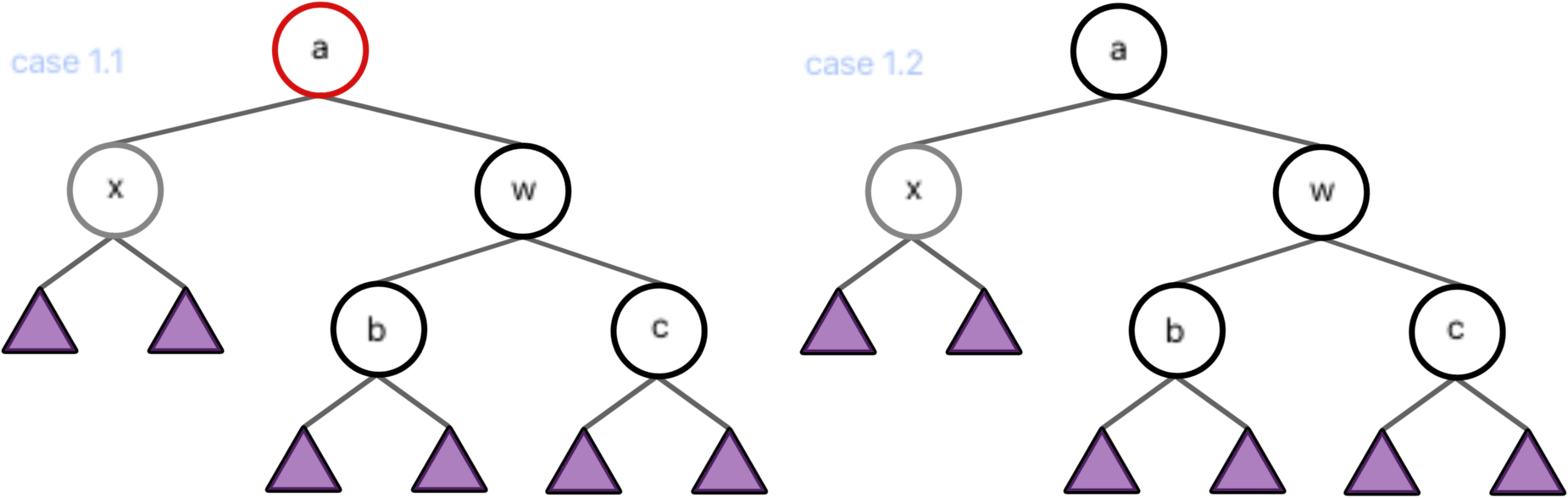Deletion / case 1.1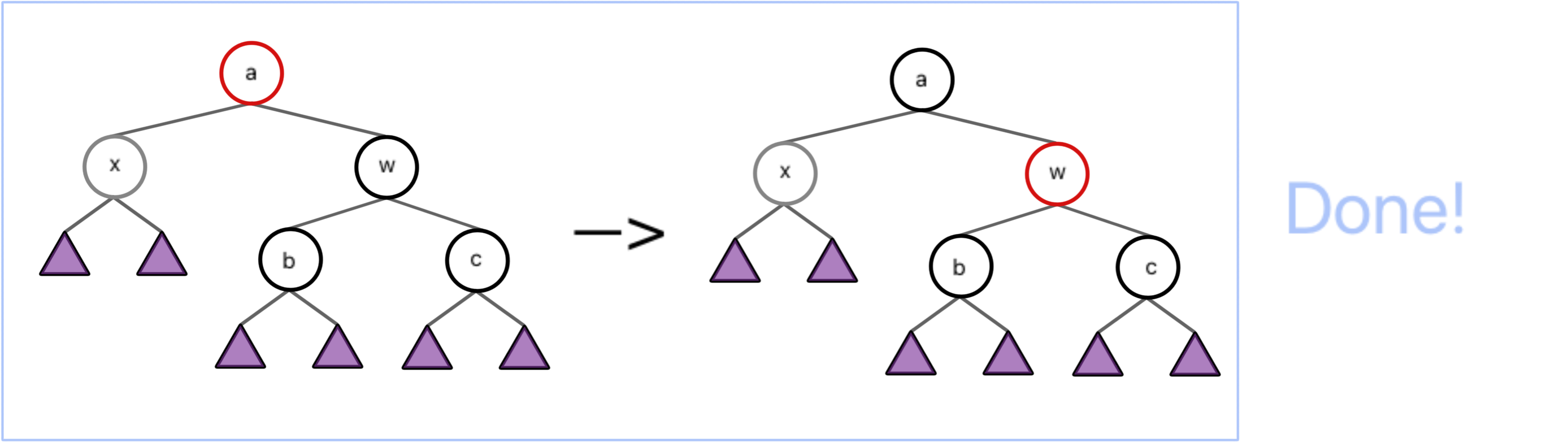Deletion / case 1.2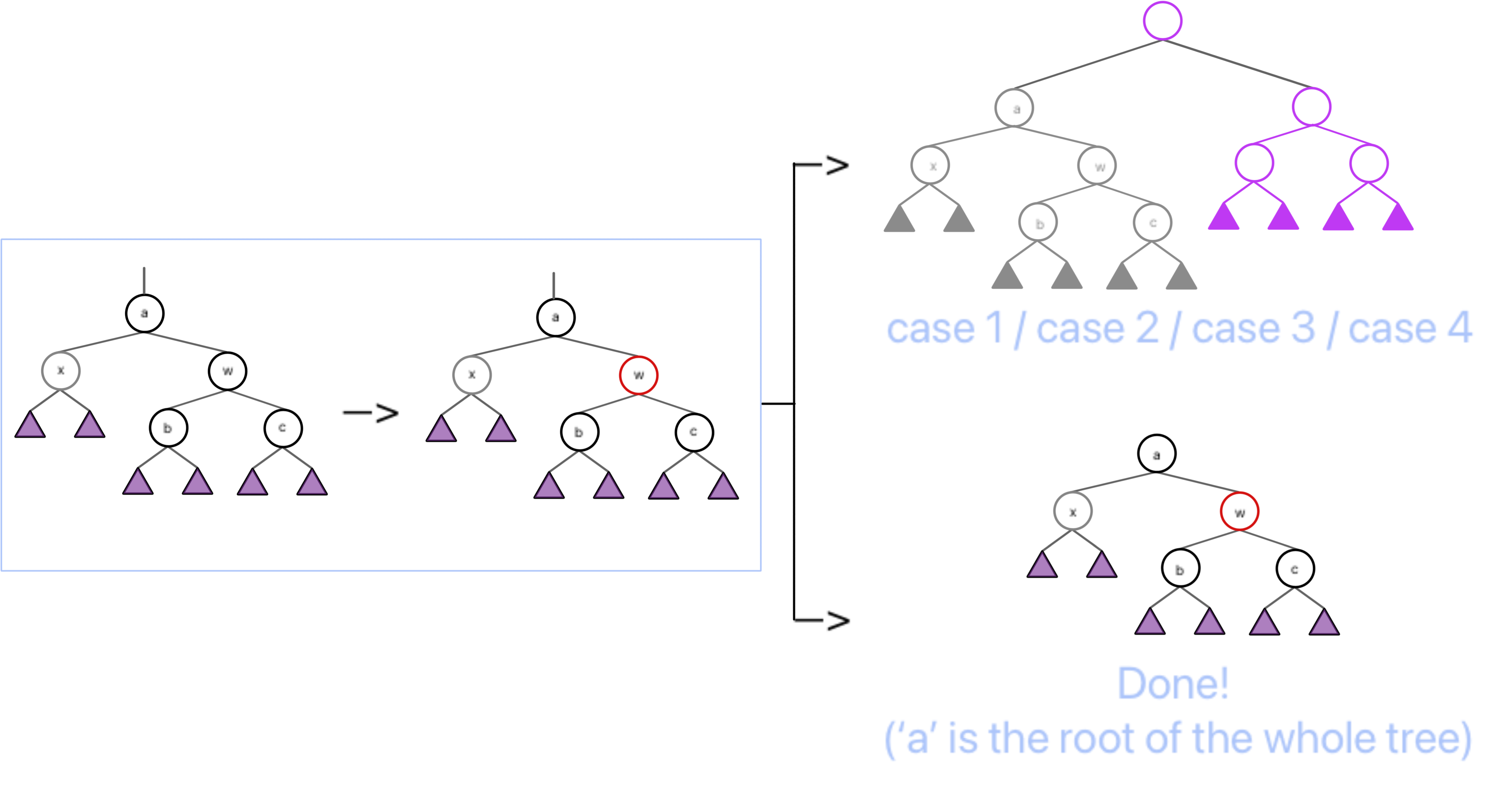Deletion / case 2

1. 将 w 染为 a 的颜色，再将 a 和 c 染成黑色；
2. 将 a 左旋，使 w 成为这个子树新的根，a 成为 w 的左儿子，b 成为 a 的右儿子；
3. 调整结束；

Deletion / case 3

1. 交换 b 和 w 的颜色；
2. 将 w 右旋，使 b 成为 a 的右儿子，w 成为 b 的右儿子，b 的右儿子成为 w 的左儿子；
3. 此时情况转化为 case 2；

Deletion / case 4

1. 交换 a 和 w 的颜色；
2. 将 a 左旋，使 w 成为这个子树新的根，a 成为 w 的左儿子，b 成为 a 的右儿子；
3. 此时根据子树 a 的情况，转化为 case 1.1 / case 2 / case 3；
graph LR;
A1["case 1.1"]
A2["case 1.2"]
B["case 2"]
C["case 3"]
D["case 4"]
E["finish"]

A1 --->|"C"| E
A2 ===>|"C"| A1
A2 ===>|"C"| A2
A2 ===>|"C"| B
A2 ===>|"C"| C
A2 ===>|"C"| D
C --->|"C&R"| B --->|"C&R"| E
D ===>|"C&R"| A1
D ===>|"C&R"| B
D ===>|"C&R"| C
graph LR;
A["case 1"]
B["case 2"]
C["case 3"]
D["case 4"]
E["finish"]

A --->|"C\nfrom case 1.1"| E
A ===>|"C\nfrom case 1.2"| A
A ===>|"C\nfrom case 1.2"| B
A ===>|"C\nfrom case 1.2"| C
A ===>|"C\nfrom case 1.2"| D
C --->|"C&R"| B --->|"C&R"| E
D ===>|"C&R\nto case 1.1 "| A
D ===>|"C&R"| B
D ===>|"C&R"| C

Option AVL Tree RB Tree
Insertion $$\leq 2$$ $$\leq 2$$
Deletion $$O(\log N)$$ $$\leq 3$$

## B+ Tree¶

### 概念¶

B+ 树是一种用树状形式维护有序数列比较信息的数据结构，其增改操作拥相对于二叉树结构更加稳定的对数时间复杂度，通常用于数据库和操作系统的文件系统中。

B+ Treeproperty of B+ Tree

@cy's PPT

1. The root is either a leaf or has between $$2$$ and $$M$$ children.
2. All nonleaf nodes (except the root) have between $$\lceil M/2 \rceil$$ and M children.
3. All leaves are at the same depth.

Assume each nonroot leaf also has between $$\lceil M/2 \rceil$$ and $$M$$ children.

depth of B+ Tree

### 操作¶

#### 查找¶Find(43)#### 插入¶

Insert(46), no splitInsert(44), split